# Magneto-electro-mechanical size-dependent vibration analysis of three-layered nanobeam with initial curvature considering thickness stretching

Document Type : Reasearch Paper

Author

Department of Solid Mechanics, Faculty of Mechanical Engineering, University of Kashan, Kashan 87317-51167, Iran.

Abstract

Thickness stretching effect based on shear and normal deformation theory is used in this paper for magneto-electro-elastic vibration analysis of a three-layered curved nanobeam including a nano core and two piezo-magnetic layers. Size-dependency is included in derivation of governing equations of motion based Eringen's nonlocal elasticity theory. The initial curvature is accounted in calculation of external works due to pre-mechanical, electrical and magnetic loads. The analytical method is presented to study the effect of significant parameters on the vibration characteristics. The numerical results are presented in terms of initial electro-magneto-mechanical loads, size-dependency parameter, opening angle, two parameters of Pasternak's foundation and core thickness to face-sheet thickness ratio.

Keywords

Main Subjects

#### Full Text

INTRODUCTION

Shear deformation theories have been developed to model kinematic relations in various structures such as beams, plates and shells. Most of these theories assumed that transverse deflection across the thickness direction is constant and do not varies with change of thickness coordinate. These limitation leads to inaccurate results for thick walled shells, plates and beams. Shear and normal deformation theory has been proposed to calculate thickness stretching for more accurate calculation of deformations and stresses across the thickness direction. This theory considers thickness stretching through employing a function for transverse function in terms of thickness coordinate. In this paper, magneto-electro-elastic vibration analysis of a three-layered curved nano beam is presented based on nonlocal elasticity theory and shear and normal deformation theory. One can conclude that combination of this topic with some non-classical theories such nonlocal elasticity theory leads to significant issue in size-dependent analysis of structures. Literature review is presented here based on published works on the shear and normal deformation theory, nonlocal elasticity theory and curvilinear coordinate system.

Zenkour  presented an analytical work on the bending analysis of cross-ply laminated and sandwich beams based on higher-order shear deformation theory accounting shear and normal deformations. The numerical results were presented for simply-supported beam in terms of important parameters of the problem. Arefi and Zenkour  used a simplified shear and normal deformations nonlocal theory for bending analysis of functionally graded piezomagnetic sandwich nanobeams in magneto-thermo-electric environment. The influence of magneto-electro-thermal loads was studied on the bending results of nanobeam. Shi  analyzed bending behaviors of a piezoelectric and functionally graded curved actuator based on theory of piezo-elasticity subjected to an external voltage. The influence of power index of functionally graded material was investigated on the results and the obtained results were approved by comparison with finite element approach. Koutsawa and Daya  presented static and free vibration analyses of laminated glass beam rest on viscoelastic foundation based on finite element method. Qian et al.  presented static, free and forced vibration analysis of thick rectangular functionally graded elastic plate based on higher-order shear and normal deformation plate theory. The problem was solved using a meshless local Petrov–Galerkin method. Bending analysis of a functionally graded piezoelectric curved beam subjected to external electric potential was studied by Shi and Zhang . Theory of piezo-elasticity was employed for derivation of the governing equations of the model and the bending results were derived using Taylor series expansion method. Belabed et al.  employed a simple higher-order shear and normal deformation theory for dynamic analysis of functionally graded plates. Based on this theory, the transverse displacement is divided to three parts including bending, shear and thickness stretching parts. A hyperbolic variation was assumed for thickness stretching function to overcome limitation of other theories and satisfies free stress boundary conditions on top and bottom of plate. The numerical results were compared with those results using three dimensional and quasi three dimensional solutions. Zhou et al.  studied the transient analysis of a curved piezoelectric beam with variable curvature as piezoelectric vibration energy harvester. Bousahla et al.  studied the influence of stretching effect on the static analysis of functionally graded composite plates based on a trigonometric higher-order shear and normal deformation theory. The concept of neutral surface was included in the derivation procedure. They concluded that employing the concept of neutral surface effect on the formulation procedure eliminates stretching–bending coupling effect and reduces the governing equations to the simple form of those derived for isotropic materials. Hajianmaleki et al.  presented a complete review on the vibration analysis of straight and curved laminated composite beams based on various analytical and numerical methods such as shear deformation theory and finite element method, respectively. Rahimi et al.  studied electro-elastic analysis of functionally graded piezoelectric material cylindrical shell. The effect of electric potential was studied on the bending results.

Some important studies on piezo-magnetic analysis of structures can be observed in references [23-24]. Ebrahimi and Barati  employed nonlocal elasticity to study the buckling behavior of curved magneto-electro-elastic FG nanobeams based on principle of virtual work and Euler-Bernoulli beam theory. The gradation of material properties was considered based on power-law function along the thickness direction. One can conclude that although references [21, 24] mentioned buckling analysis of curved beam, however the influence of electro-magnetic loads of curved nano beam on the bending behaviors of structure has not been performed by the same and other researchers. Nonlinear vibration analysis of functionally graded porous micro/nano-plates reinforced with graphene nanoplates was studied by Sahmani et al.  based on nonlocal strain gradient theory. The von-Karman nonlinear strains were included in kinematic relations. Larbi et al.  studied bending and free vibration analysis of functionally graded beams based on shear and normal deformation beam theory and physical neutral surface effect. A hyperbolic function was used for distribution of shear stress across thickness direction. Yu et al.  presented elastic analysis of initially curved and twisted anisotropic beams based on three-dimensional elasticity theory and Timoshenko theory. Nonlocal elasticity was used for buckling and free vibration analysis of nanosheets , single walled carbon nanotubes  and non-uniform nanobeam . Ghasemi et al. [31-32] presented some computational design methodology for topology optimization of multi-material-based flexoelectric composites and a design methodology based on a combination of isogeometric analysis, respectively. Hamida et al.  studied the sensitivity analysis of a flexoelectric nanostructure based on NURBS-based IGA formulation. The numerical results indicated that the flexoelectric constants are the most dominant factors influencing the uncertainties in the energy conversion factor. The effect of flexoelectricity was studied on the topology optimization and dynamic responses of flexoelectric nanostructures [34-35].

The literature review was completed by considering the reports including various analysis of curved structures and application of shear and normal deformation theory to various analysis of beams and plates. This review indicates that although some important works on the curved beams and application of shear and normal deformation theory to various analysis of the beams have been published, however it can be strongly verified that there is no published work about size-dependent magneto-electro-elastic vibration analysis of three-layered curved nanobeams subjected to mechanical, electrical and magnetic loads resting on Pasternak’s foundation. To account thickness stretching effect and size-dependency, the shear and normal deformation theory and Eringen nonlocal elasticity theory are used respectively. The governing equations of motion are derived based on the Hamilton’s principle. The effect of initial magneto-electro-mechanical loads is investigated on the natural frequencies of three-layered curved nanobeam.

MATERIALS AND METHODS

Shear and normal deformation theory is used to accounts thickness stretching for magneto-electro-elastic formulation of a three-layered curved nanobeam (Fig. 1) subjected to magneto-electro-mechanical loads. Based on this theory, we will have displacement field as follows: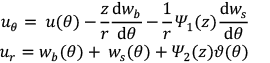(1)

where u(θ) is displacement of the middle-surface along transverse direction; wb and ws are the bending and shear components of the radial displacement ui, and θ is a function that accounts thickness stretching as function of ϑ. In addition, r is local radius and z is measures from middle surface in which the relation between them is expressed as: r=R+z (Fig. 1). The above displacement field shows that the term Ψ2 (z)ϑ(θ) is applied to account thickness stretching in displacement field. The shape functions associated with refined shear and normal deformation curved beam theory are presented as [1, 2]: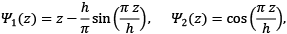(2)

The normal and shear strain components are expressed as: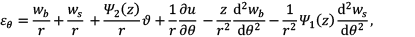(3)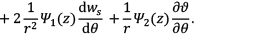The strain components are considered for both core and piezomagnetic sections. It is assumed that piezomagnetic layers are completely attached to core with no discontinuity. Based on this assumption, the displacement filed is assumed continuous between core and piezomagnetic layers. In addition, it is assumed that piezomagntic layers are subjected to intial electric and magnetic potentials along the thickness direction.

The constitutive relations for isotropic core are defined as :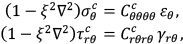(4)

in which  are stiffness coefficients of elastic core, ∇ 2 is Laplace operator in polar coordinate system and ξ=e0 a is nonlocal parameter. Furthermore, the constitutive relations for piezomagnetic layers are defined as [6, 15, 19]: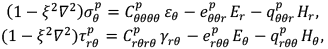(5)

in which  are stiffness coefficients of piezoelectric layers,  are the piezoelectric coefficients and  are piezomagnetic coefficients. Ei and Hi are the components of electric and magnetic fields, respectively that are defined as [15-19]: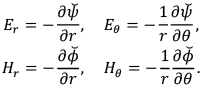(6)

The electric and magnetic potentials are assumed as [15-19]:

In which ψ00 are applied electric and magnetic potentials,  for top and bottom piezo-magnetic face-sheets. Substitution of electric and magnetic potentials from Eq. (7) into Eq. (6) gives electric and magnetic fields as follows:

The electric displacement and magnetic induction along the radial and circumferential directions are derived as [15-19]:

in which mij and μij are dielectric and electromagnetic coefficients. Substitution of strain, electric and magnetic fields into constitutive relations leads to following relations for core and piezomagnetic layers as:

in which the integration constants A1, A2,… are expressed in the Appendix. In addition, the variation of energy due to external works is given by

in which Rf is reaction of Pasternak’s foundation. Note that in spite of all previous papers, the external work by multiplication of reaction of foundation and uniform load in transverse deformation of bottom and top, respectively. The reaction of Pasternak’s foundation is defined as:

where K1 and K2are spring and shear parameters of foundation. In addition, the variation of work due to initial electric and magnetic potentials is calculated as follows: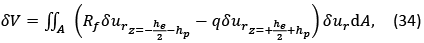(36)

In which N0.NE.NM are mechanical, electrical and magnetic pre-loads. These preloads are defined as: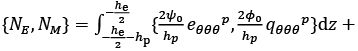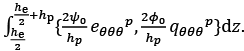(37)

In addition, variation of kinetic energy is defined as follows: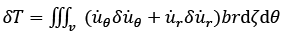(38)

By substitution of displacement field into Eq. (37) and integration by part and then definition of integration of constants, we will have variation of kinetic energy as follows:

RESULTS AND DISCUSSIONS

In this section, the solution procedure for free vibration analysis is developed. The proposed solutions for a simply-supported three-layered curved nanobeam are expressed as: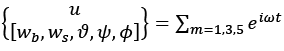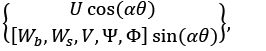(52)

in which α=mπR/L. Substitution of proposed solution into governing equations of motion leads to below equation: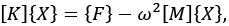(53)

in which  is an unknown vector corresponding to five unknown functions. The symmetric elements of the matrix [K], [M] are expressed as:

In this section, the numerical results of the problem are presented. Before presentation of numerical results, the material properties of elastic core and piezomagnetic layers should be introduced .

Core:

Piezomagnetic layers :In continuation, the responses of three-layered curved nanobeam due to initial magneto-electro-mechanical loads are investigated. The vibration responses are including 1st, 2nd and 3rd natural frequencies. Table 1 lists variation of 1st, 2nd and 3rd natural frequencies of three-layered curved nanobeam subjected to initial electric potential Ψ0. The numerical results indicate that with increase of initial electric potential Ψ0 the natural frequencies of three-layered curved nanobeam are increased significantly. It is concluded that with increase of initial electric potential, the electrical pre-load of curved nanobeam is increased and then the natural frequencies are increased. Furthermore, this conclusion is in accordance with results of Reference [28, 29].

The influence of initial magnetic potential Φ0 on the natural frequencies of three-layered curved nanobeam is listed in Table 2. The numerical results show that with increase of initial magnetic potential, the natural frequencies are decreased significantly. One can conclude that the pre-load of curved nanobeam is decreased with increase of initial magnetic potential in accordance with results of Reference .

The influences of initial mechanical loads on the natural frequencies of three-layered curved nanobeam are presented in Table 3. One can conclude that increase of initial mechanical loads leads to decrease of stiffness and consequently decrease of natural frequencies.

In continuation, the influence of small scale parameter and two parameters of Pasternak’s foundation is studied on the natural frequencies of three-layered curved nanobeam. The influence of nonlocal parameters ξ are studied on the natural frequencies of three-layered curved nanobeam in Table 4. Table 4 lists variation 1st, 2nd and 3rd natural frequencies of three-layered curved nanobeam in terms of various nonlocal parameters. One can conclude that with increase of nonlocal parameter, the stiffness of nano materials is decreased and consequently the natural frequencies are decreased . Tables 5, 6 list variation of 1st, 2nd and 3rd natural frequencies of three-layered curved nanobeam in terms of spring K1and shear K2 parameters of foundation, respectively. It is observed the natural frequencies are increased with increase of spring K1and shear K2 parameters of foundation. It can be concluded that with increase of spring and shear parameters of Pasternak’s foundation, the stiffness of structure is increased and then the natural frequencies are increased significantly.

To study the influence of thickness of core and piezoelectric on the natural frequencies of three-layered curved nanobeam, the non-dimensional core thickness to piezoelectric thickness ratio  is employed for case the total thickness is assumed fixed (he+2hp=Const). Shown in Fig. 2 is variation of 1st, 2nd and 3rd natural frequencies of three-layered curved nanobeam in terms of core thickness to piezoelectric thickness ratio. The numerical results show that with increase of this parameter, the natural frequencies are increased significantly. It is concluded that with increase of core thickness to piezoelectric thickness ratio, the portion of core is increased rather than the portion of core in bending stiffness of nanobeam that leads to increase of natural frequencies.

Shown in Fig. 3 is variation of 1st, 2nd and 3rd natural frequencies of three-layered curved nanobeam in terms of various opening angles. The numerical results show interesting behavior for fundamental and higher-order natural frequencies. It is concluded that the fundamental natural frequencies are decreased with increase of opening angle while the 2nd and 3rd natural frequencies are increased with increase of opening angle.

CONCLUSIONS

Magneto-electro-elastic vibration characteristics of a three-layered curved nanobeam resting on Pasternak’s foundation was studied in this paper. Thickness stretching effect was included in the governing equations of motion based on shear and normal deformation theory. The shear and normal deformation theory used a sinusoidal distribution of shear stress across the thickness direction. Size dependency was accounted based on Eringen’s nonlocal elasticity theory. The governing equations of motion were derived based on Hamilton’s principle. The analytical method was presented parametrically based on Navier’s method for simply supported curved nanobeam. The numerical results were presented to show the influence of nonlocal parameter, opening angle, the core thickness to piezoelectric thickness ratio on the vibration characteristics of three-layered curved nanobeam. The numerical results present some important conclusions as follows:

- The three-layered curved nanobeam was subjected to initial mechanical, electrical and magnetic loads. The numerical results indicate that these initial loads can strongly affect the vibration characteristics of curved nanobeam. One can conclude that the natural frequencies are increased with increase of initial electric potential ψ0. In addition, increase of initial mechanical loads N0 and magnetic potential ϕ0 leads to decrease of natural frequencies of three-layered curved nanobeam.

- The nonlocal parameter ξ based on Eringen’s nonlocal elasticity theory leads to change of natural frequencies of curved nanobeam. One can conclude that increase of nonlocal parameter decreases the stiffness of nanobeam and decrease of natural frequencies.

- The results were presented in terms of geometric parameters such opening angle and the core thickness to piezoelectric thickness ratio. The numerical results indicate that the fundamental natural frequencies are decreased with increase of opening angle while the 2nd and 3rd natural frequencies are increased with increase of opening angle. In addition, increase of the core thickness to piezoelectric thickness ratio leads to increase of natural frequencies due to increase of stiffness.

APPENDIX

CONFLICT OF INTEREST

The authors declare that there is no conflict of interests regarding the publication of this review article.

#### References

1. Zenkour A. M., (1999), Transverse shear and normal deformation theory for bending analysis of laminated and sandwich elastic beams. Mech. Compos. Mater. Struc. 6: 267-283.
2. Arefi M., Zenkour A. M., (2016), A simplified shear and normal deformations nonlocal theory for bending of functionally graded piezomagnetic sandwich nanobeams in magneto-thermo-electric environment. J. Sandw. Struct. Mater. 18: 624-651.
3. Shi Z. F., (2005), Bending behavior of piezoelectric curved actuator. Smart. Mater. Struct. 14: 835–842.
4. Koutsawa Y., Daya E. M., (2007), Static and free vibration analysis of laminated glass beam on viscoelastic supports. Int. J. Solids. Struct. 44: 8735–8750.
5. Qian L. F., Batra R. C., Chen L. M., (2004), Static and dynamic deformations of thick functionally graded elastic plates by using higher-order shear and normal deformable plate theory and meshless local Petrov–Galerkin method. Compos. Part B: Eng. 35: 685-697.
6. Shi Z. F., Zhang T., (2008), Bending analysis of a piezoelectric curved actuator with a generally graded property for the piezoelectric parameter. Smart. Mater. Struct 17: 045018-045022.
7. Belabed Z., Houari M. S. A., Tounsi A., Mahmoud S. R., Anwar Bég O., (2014), An efficient and simple higher order shear and normal deformation theory for functionally graded material (FGM) plates. Compos. Part B: Eng. 60: 274-283.
8. Zhou Y., Nyberg T. R., Xiong G., Zhou H., Li S., (2016), Precise deflection analysis of laminated piezoelectric curved beam. J. Intel. Mater. Syst. Struct. 27: 2179-2198.
9. Bousahla A. A., Houari M. S. A. Tounsi A., Addabedia E. A., (2014), A Novel higher order shear and normal deformation theory based on neutral surface position for bending analysis of advanced composite plates. Int. J. Comput. Methods. 11: 1350082-1350085.
10. Hajianmaleki M., Qatu M. S., (2013), Vibrations of straight and curved composite beams: A review. Compos. Struct. 100: 218–232.
11. Rahimi G. H., Arefi M., Khoshgoftar M. J., (2012), Electro elastic analysis of a pressurized thick-walled functionally graded piezoelectric cylinder using the first order shear deformation theory and energy method, Mechanika. 18: 292-300.
12. Arslan E., Usta R., (2014), Mechanical and electrical ﬁelds of piezoelectric curved sensors. Arch. Mech. 66: 329–342.
13. Arefi M., (2015), Elastic solution of a curved beam made of functionally graded materials with different cross sections. Steel Compos. Struct. 18: 659-672.
14. Houari M. S. A., Tounsi A., Bégc O. A., (2013), Thermoelastic bending analysis of functionally graded sandwich plates using a new higher order shear and normal deformation theory. Int. J. Mech. Sci. 76: 102-111.
15. Arefi M., Zenkour A. M., (2016), Free vibration, wave propagation and tension analyses of a sandwich micro/nano rod subjected to electric potential using strain gradient theory. Mater. Res. Exp. 3: 115704-115709.
16. Arefi M., (2016), Surface effect and non-local elasticity in wave propagation of functionally graded piezoelectric nano-rod excited to applied voltage. Appl. Math. Mech. 37: 289-302.
17. Arefi M., Zenkour A. M., (2017), Wave propagation analysis of a functionally graded magneto-electro-elastic nanobeam rest on Visco-Pasternak foundation. Mech. Res. Com. 79: 51-62.
18. Arefi M., Zenkour A. M., (2017), Effect of thermo-magneto-electro-mechanical fields on the bending behaviors of a three-layered nanoplates based on sinusoidal shear-deformation plate theory. J. Sandw. Struct. Mater. In Press, Doi:1099636217697497.
19. Arefi M., (2014), A complete set of equations for piezo-magnetoelastic analysis of a functionally graded thick shell of revolution. Lat. Amer. J. Solids. Struct. 11: 2073-2092.
20. Bourada M., Kaci A., Houari M. S. A., Tounsi A., (2015), A new simple shear and normal deformations theory for functionally graded beams. Steel. Compos. Struct. 18: 409-423.
21. Natarajan S., Chakraborty S., Thangavel M., Bordas S., Rabczuk T., (2012), Size-dependent free flexural vibration behavior of functionally graded nanoplates. Comput. Mater. Sci. 65: 74-80.
22. Bennai R., Atmane H. A., Tounsi A., (2015), A new higher-order shear and normal deformation theory for functionally graded sandwich beams. Steel. Compos. Struct. 19: 521-546.
23. Hou P. F., Leung A. Y. T., (2004), The transient responses of magneto-electro-elastic hollow cylinders. Smart. Mater. Struct. 13: 762–776.
24. Ebrahimi F., Barati M. R., (2016), Magneto-electro-elastic buckling analysis of nonlocal curved nanobeams. Eur. Phys. J. Plus. 131: 346-351.
25. Sahmani S., Mohammadi Aghdam M., Rabczuk T., (2018), Nonlocal strain gradient plate model for nonlinear large-amplitude vibrations of functionally graded porous micro/nano-plates reinforced with GPLs. Compos. Struct. 198: 51-62.
26. Larbi L. O., Kaci A. M., Ahmed S., (2013), An efficient shear deformation beam theory based on neutral surface position for bending and free vibration of functionally graded beams. Steel. Compos. Struct. 41: 23-48
27. Yu W., Hodges D. H., Volovoi V., Cesnik C. E. S., (2002), On timoshenko-like modeling of initially curved and twisted composite beams. Int. J. Solids. Struct. 39: 5101–5121.
28. Tourki Samaei A., Hosseini Hashemi Sh., (2012), Buckling analysis of graphene nanosheets based on nonlocal elasticity theory. Int. J. Nano Dimens. 2: 227-232.
29. Ansari R., Rouhi H., (2015), Nonlocal flügge shell model for the axial buckling of single-walled Carbon nanotubes: An analytical approach. Int. J. Nano Dimens. 6: 453-462.
30. Hosseini Hashemi Sh., Bakhshi Khaniki H., (2017), Vibration analysis of a Timoshenko non-uniform nanobeam based on nonlocal theory: An analytical solution. Int. J. Nano Dimens. 8: 70-81.
31. Ghasemi H., Park H. S., Rabczuk T., (2018), A multi-material level set-based topology optimization of flexoelectric composites. Comput. Meth. Appl. Mech. Eng. 332: 47-62.
32. Ghasemi H., Park H. S., Rabczuk T., (2017), A level-set based IGA formulation for topology optimization of flexoelectric materials. Comput. Meth. Appl. Mech. Eng. 313: 239-258.
33. Hamdia K. M., Ghasemi H., Zhuang X., Alajlan N., Rabczuk T., (2018), Sensitivity and uncertainty analysis for flexoelectric nanostructures. Comput. Meth. Appl. Mech. Eng. 337: 95-109.
34. Nanthakumar S. S., Zhuang X., Park H. S., Rabczuk T., (2017), Topology optimization of flexoelectric structures. J. Mech. Phys. Solids. 105: 217-234.
35. Nguyen B. H., Nanthakumar S. S., Zhuang X., Wriggers P., Rabczuk T., (2018), Dynamic flexoelectric effect on piezoelectric nanostructures. Eur. J. Mech. A/Solids. 71: 404-409.
36. Hou P. F., Leung A. Y. T., (2004), The transient responses of magneto-electro-elastic hollow cylinders. Smart. Mater. Struct. 13: 762–776.